# Fit with half-normal plateau detection function and home range size estimation using nimbleSCR package

#### 2022-11-30

In this vignette, we demonstrate how to use the nimbleSCR (Bischof et al. 2020) and NIMBLE (Valpine et al. 2017; NIMBLE Development Team 2021) packages to simulate and fit a Bayesian SCR model with a half-normal plateau detection function and derive home-range size estimates from the estimated parameters (Dey et al. (2022)). We use a method based on numerical approximation to estimate home range size from SCR models with circular detection functions.

# LOAD PACKAGES
library(coda)
library(nimble)
library(nimbleSCR)
library(basicMCMCplots)

## 1. Binomial SCR Model with half-normal plateau detection function

### 1.1 Habitat and trapping grid

For this example, we create a $$30 \times 30$$ habitat grid represented by grid cell centers. We center a $$20 \times 20$$ trapping grid in the habitat leaving an unsampled buffer width of 5 distance units (du) around it.

# CREATE HABITAT GRID
coordsHabitatGridCenter <- cbind(rep(seq(29.5, 0.5, by=-1), 30),
sort(rep(seq(0.5, 29.5, by=1), 30)))
colnames(coordsHabitatGridCenter) <- c("x","y")

# CREATE TRAP GRID
trapCoords <- cbind(rep(seq(5.5, 24.5,by=1),20),
sort(rep(seq(5.5, 24.5,by=1),20)))
colnames(trapCoords) <- c("x","y")

# PLOT CHECK
plot(coordsHabitatGridCenter[,"y"] ~ coordsHabitatGridCenter[,"x"], pch = 1, cex = 1.5) #pch=16)
points(trapCoords[,"y"] ~ trapCoords[,"x"], col="red", pch=16 )
par(xpd=TRUE)
legend(x = 7, y = 33.3,
legend=c("Habitat grid centers", "Traps"),
pt.cex = c(1.5,1),
horiz = T,
pch=c(1,16),
col=c("black", "red"),
bty = 'n')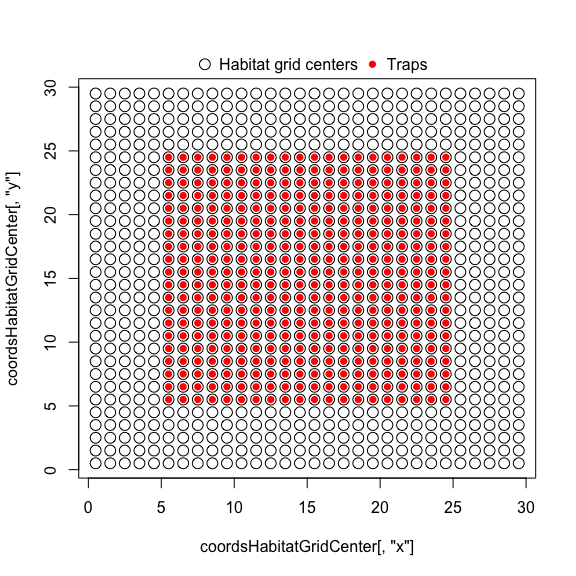In order to use nimbleSCR’s efficient functions, we have to rescale habitat and trap coordinates to to allow a fast habitat cell ID lookup and the local evaluation (see Milleret et al. (2019) and Turek et al. (2021)
for further details). We use the ‘scaleCoordsToHabitatGrid’ function to rescale coordinates and the ‘getLocalObjects’ function to set up the objects necessary to perform the local evaluation. Special care should be taken when chosing ‘dmax’, the radius within which traps are considered, relative to $$\sigma$$ (Milleret et al. 2019). Here, we are using a value $$>2 * \sigma$$.

## RESCALE COORDINATES
ScaledCoords <- scaleCoordsToHabitatGrid(coordsData =  trapCoords,
coordsHabitatGridCenter = coordsHabitatGridCenter)

lowerAndUpperCoords <- getWindowCoords(
scaledHabGridCenter = ScaledCoords$coordsHabitatGridCenterScaled, scaledObsGridCenter = ScaledCoords$coordsDataScaled,
plot.check = F)

## LOCAL EVALUATION
habitatMask <- matrix(1, nrow = 30, ncol= 30, byrow = TRUE)

coords = ScaledCoords$coordsDataScaled, dmax = 10, plot.check = FALSE) ### 1.2 Define SCR model with half-normal plateau detection function Here, we use a custom distribution ‘dbinomLocal_normalPlateau()’ (available in the nimbleSCR package) corresponding to the half-normal plateau detection function (Dey et al. 2022). Note that we do not provide ‘detNums’ and ‘detIndices’ arguments in the ‘dbinomLocal_normalPlateau()’ function as we want to use this model to simulate data (see ‘?dbinomLocal_normalPlateau’ for further details). When simulating detections using this formulation of the SCR model in NIMBLE, all the information about detections (where and how many) is stored for each indiidual in the observation vector ‘y’ in the following order: • ‘detNums’ (total number of individual detections), • ‘x’ (number of individual detections at each trap), • ‘detIndices’ (id of the trap at which detections occur). We now need to provide the maximum number of spatial recaptures that can be simulated per individual. We recommend using ‘trapLocal$numlocalindicesmax’ that defines the maximum number of traps available for detections when local evaluation is used. This will enable the simulation of as many spatial detections as allowed by the restrictions imposed by the local evaluation (defined by the ‘dmax’ argument from ‘getLocalObjects’). This means that the length of the ‘y’ observation vector for each individual is equal to the length of $$c(detNums, x, detIndices)$$ and is therefore equal to $$lengthYCombined = 1+ 2 * trapLocal\numLocalIndicesMax$$.

lengthYCombined <- 1 + 2*trapLocal$numLocalIndicesMax  This means that: ‘y’ denotes the number of detections, ‘y[2:(trapLocal$numLocalIndicesMax+1)]’ gives the number of detections in each detector where it was detected (and ‘-1’ at the remaining indices) and ‘y[(trapLocal$numLocalIndicesMax+2):(2*trapLocal$numLocalIndicesMax+1)]’ gives the id of the traps at which detections occur (and ‘-1’ at the remaining indices).

The nimble model code of an SCR model with half-normal plateau detection function is given below.

modelCode <- nimbleCode({
## priors
psi ~ dunif(0, 1)
sigma ~ dunif(0, 50)
w ~ dunif(0, 20)
p0 ~ dunif(0, 1)

## loop over individuals
for(i in 1:M) {
## AC coordinates
sxy[i, 1:2] ~ dbernppAC(
lowerCoords = lowerHabCoords[1:numHabWindows, 1:2],
upperCoords = upperHabCoords[1:numHabWindows, 1:2],
logSumIntensity = logSumHabIntensity,
habitatGrid = habitatGrid[1:numGridRows,1:numGridCols],
numGridRows =  numGridRows,
numGridCols = numGridCols
)

z[i] ~ dbern(psi)
## likelihood
y[i, 1:lengthYCombined] ~ dbinomLocal_normalPlateau(
size = trials[1:n.traps],
p0 = p0,
s = sxy[i,1:2],
sigma = sigma,
w = w,
trapCoords = trapCoords[1:n.traps,1:2],
localTrapsIndices = trapIndex[1:n.cells,1:maxNBDets],
localTrapsNum = nTraps[1:n.cells],
habitatGrid = habitatIDDet[1:y.maxDet,1:x.maxDet],
indicator = z[i],
lengthYCombined = lengthYCombined)
}
## derived quantity: total population size
N <- sum(z[1:M])
})

### 1.3 Set parameter values to simulate

Here, we will use nimble’s functionalities to simulate a SCR data set directly from the model. For this, we need to set the values of the top-level parameters of the model:

• p0: the baseline detection probability
• sigma: the scale parameter
• w: the width of the plataue
• psi: proportion of available individuals present in the population
# PARAMETERS
p0 <- 0.25
sigma <- 1
w <- 1.5
psi <- 0.5

The model formulation uses data augmentation to derive N estimates (Royle and Dorazio 2012). We therefore need to choose the total number of individuals M (detected + augmented). Here we used 500. The expected total number of individuals present in the population is ‘M * psi’.

M <- 500

### 1.4 Create data, constants and inits objects

We can now create the lists of input data and constants required by NIMBLE to build the model.

nimConstants <- list(M = M,
n.traps = dim(ScaledCoords$coordsDataScaled), y.maxDet = dim(trapLocal$habitatGrid),
x.maxDet = dim(trapLocal$habitatGrid), n.cells = dim(trapLocal$localIndices),
maxNBDets = trapLocal$numLocalIndicesMax, nTraps = trapLocal$numLocalIndices,
lengthYCombined = lengthYCombined,
numHabWindows = dim(lowerAndUpperCoords$upperHabCoords), numGridRows = dim(lowerAndUpperCoords$habitatGrid),
numGridCols = dim(lowerAndUpperCoords$habitatGrid) ) habIntensity = rep(1, dim(lowerAndUpperCoords$upperHabCoords))
logSumHabIntensity <- log(sum(habIntensity))
logHabIntensity <- log(habIntensity)

nimData <- list(trapCoords = ScaledCoords$coordsDataScaled, trapIndex = trapLocal$localIndices,
lowerHabCoords = lowerAndUpperCoords$lowerHabCoords, upperHabCoords = lowerAndUpperCoords$upperHabCoords,
habitatGrid = lowerAndUpperCoords$habitatGrid, logHabIntensity = logHabIntensity, logSumHabIntensity = logSumHabIntensity, habitatIDDet = trapLocal$habitatGrid,
trials = rep(1, dim(ScaledCoords$coordsDataScaled)) ) In order to simulate data from the nimble model, we need to provide simulated values as initial values. nimInits <- list(p0 = p0, psi = psi, sigma = sigma, w = w) ### 1.5 Create NIMBLE model we can then build the nimble SCR model object: model <- nimbleModel( code = modelCode, constants = nimConstants, data = nimData, inits = nimInits, check = F, calculate = F)  ### 1.6 Simulate SCR data from the NIMBLE model We first need to obtain the list of nodes that will be simulated. We used the ‘getDependencies’ function from NIMBLE. Using the ‘simulate’ function from NIMBLE, we will then simulate the activity center (AC) locations (‘sxy’), the state of the individual (‘z’) and SCR observation data (‘y’) given the values we provided for ‘p0’, ‘sigma’, ‘w’ and ‘psi’. # FIRST WE GET THE NODES TO SIMULATE nodesToSim <- model$getDependencies(c("sxy", "z"), self=T)
# THEN WE SIMULATE THOSE NODES
set.seed(1234)
model$simulate(nodesToSim, includeData = FALSE) After running ‘simulate’, the simulated data are stored in the ‘model’ object. For example, we can access the simulated ‘z’ and check how many individuals were considered present: N <- sum(model$z)

We have simulated 257 individuals present in the population of which 162 were detected.

### 1.7. MCMC with NIMBLE

#### 1.7.1 Compile the nimble model and create MCMC configuration

Here, we build the NIMBLE model again using the simulated ‘y’ as data. For simplicity, we used the simulated ‘z’ as initial values. Then we can fit the SCR model with the simulated ‘y’ data set.

nimData1 <- nimData
nimData1$y <- model$y
nimInits1 <- nimInits
nimInits1$z <- model$z
nimInits1$sxy <- model$sxy

# CREATE AND COMPILE THE NIMBLE MODEL
model <- nimbleModel( code = modelCode,
constants = nimConstants,
data = nimData1,
inits = nimInits1,
check = F,
calculate = F)

model$calculate() ##  -5721.569 MCMCconf <- configureMCMC(model = model, monitors = c("N", "sigma", "p0","w","psi"), control = list(reflective = TRUE), thin = 1) ## ===== Monitors ===== ## thin = 1: N, p0, psi, sigma, w ## ===== Samplers ===== ## binary sampler (500) ## - z[] (500 elements) ## RW_block sampler (500) ## - sxy[] (500 multivariate elements) ## RW sampler (4) ## - psi ## - sigma ## - w ## - p0 samplerConfList <- MCMCconf$getSamplers()
print(samplerConfList[1:5])
## []
## RW sampler: psi,  reflective: TRUE
## []
## RW sampler: sigma,  reflective: TRUE
## []
## RW sampler: w,  reflective: TRUE
## []
## RW sampler: p0,  reflective: TRUE
## []
## binary sampler: z,  reflective: TRUE
cmodel <- compileNimble(model)

#### 1.7.2 Update the ‘adaptive’ arguments of MCMC samplers for sigma and w

We noticed some covariation between the parameters $$\sigma$$ and $$w$$ while obtaining MCMC samples. For a better mixing of these parameters, we adjust the ‘adaptive’ arguments of the control list in MCMC samplers. We also implement repeated sampling of these two nodes within a single MCMC iteration.

# sigma
control <- samplerConfList[]$control control$log <- F
control$reflective <- T control$adaptive <- T
control$scale <- 1 samplerConfList[]$setControl(control)

# w
control <- samplerConfList[]$control control$log <- F
control$reflective <- T control$adaptive <- T
control$scale <- 1 samplerConfList[]$setControl(control)

# Use this modified list of samplerConf objects in the MCMC configuration
MCMCconf$setSamplers(samplerConfList) # Retrieve the current ordering of sampler execution ordering <- MCMCconf$getSamplerExecutionOrder()
len <- length(ordering)
MCMCconfsetSamplerExecutionOrder(c(ordering, rep(ordering, 10), # sigma rep(ordering, 10), # w ordering[4:len])) #### 1.7.3 Run MCMC Now we proceed to build and compile the nimble MCMC object. We use the function ‘runMCMC’ to execute the compiled MCMC object for obtaining posterior samples. MCMC <- buildMCMC(MCMCconf) cMCMC <- compileNimble(MCMC, project = model, resetFunctions = TRUE) # RUN THE MCMC niter <- 10000 burnin <- 2000 nchains <- 3 MCMCRuntime <- system.time(myNimbleOutput <- runMCMC( mcmc = cMCMC, nburnin = burnin, niter = niter, nchains = nchains, samplesAsCodaMCMC = TRUE)) print(MCMCRuntime) ## user system elapsed ## 5422.68 1.11 5431.26 #plot check chainsPlot(myNimbleOutput, line = c(N, p0, N/M, sigma, w))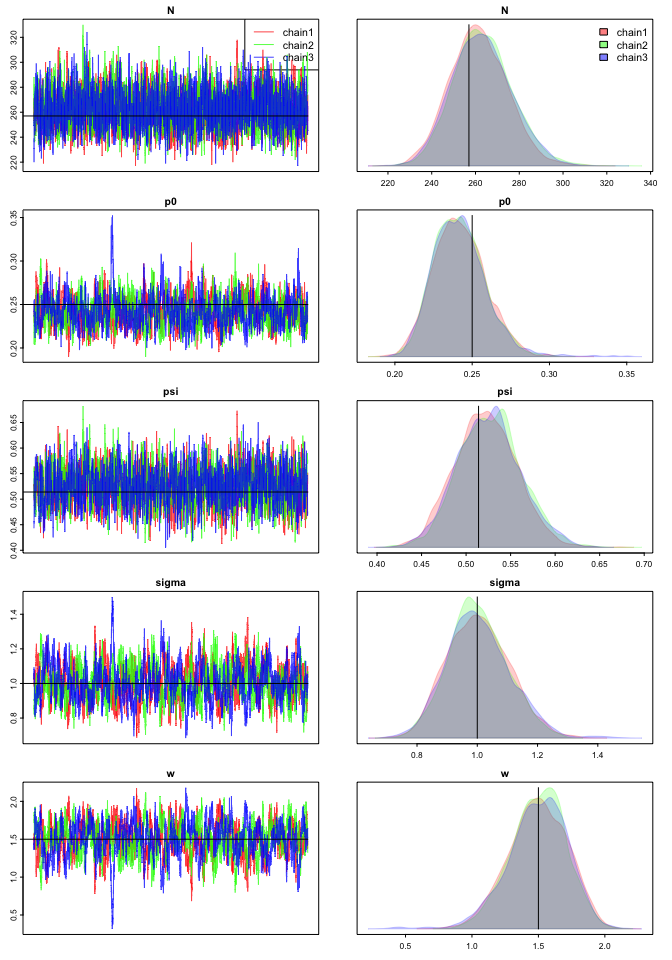## 1.8. Computation of home range size Estimating home-range size for a given circular detection function is equivalent to finding an estimate of the quantile $$r_\alpha$$ such that $$\alpha\%$$ of all movements lie within the circle of radius $$r_\alpha$$ centered on activity centre $$\mathbf{\textit{s}}$$. We can then define the $$\alpha\%$$ home range area as $$A_\alpha$$, the set of all points $$\mathbf{\textit{x}}$$ in the habitat such that $$||\mathbf{\textit{x}} − \mathbf{\textit{s}}|| ≤ r_\alpha$$. While an analytical estimate can be derived for the classical half-normal detection function (see below), the half-normal plateau is a custom detection function for which an analytical estimate $$r_\alpha$$ is not readily available. The half-normal plateau detection function is written as: \begin{align} p_{\text{HNP}}(d) = \begin{cases} p_0,& \text{if } d < w,\\[-0.5em] p_0 \, \exp\Big{(}-\frac{(d-w)^2}{2\sigma^2}\Big{)}, & \text{if } d \geq w, \end{cases} \end{align} where $$p_0 \in (0,1)$$, $$\sigma > 0$$ and $$w \geq 0$$. Here $$d$$ can be interpreted as the distance between a given location and activity centre of an animal. The parameters in the function: $$p_0$$ denotes the baseline detection probability, $$\sigma$$ denotes the scale parameter, and $$w$$ denotes the plateau length where the detection probability remains constant. For a better understanding, we also plot the detection function against distance in below. ## HALF-NORMAL PLATEAU FUNCTION detfun.HNP <- function(D, p0, sigma, w){ bool <- D <= w which_not_bool <- which(!bool) dens <- numeric(length = length(D)) dens[bool] <- 1 adjustedD2 <- (D[which_not_bool]-w)^2 dens[which_not_bool] <- exp(-(adjustedD2)/(2.0*sigma*sigma)) return(p0*dens) } par(mfrow=c(1,1)) x <- seq(0, 15, len=10001) plot(x, detfun.HNP(D=x, p0=0.2, sigma = 1, w = 1), type = "l", lwd=2, col = "orange", main = "Half-normal plateau detection function", xlab = 'd', ylab = 'Detection probability') lines(x, detfun.HNP(D=x, p0=0.1, sigma = 1.5, w = 3), lwd=2, lty = "solid", col = "darkcyan") focal.vars <- c('p0', 'sigma', 'w') focal.values <- cbind(c(0.2, 0.1), # p0 c(1, 1.5), # sigma c(1, 3)) # w legend.items <- apply(focal.values, 1, function(s){paste(paste(focal.vars,"=",s,sep=" "),collapse=", ")}) legend("topright", legend = legend.items, lty = c('solid', 'solid'), bg="transparent", col=c('orange', "darkcyan"), cex = 0.75, bty = 'n')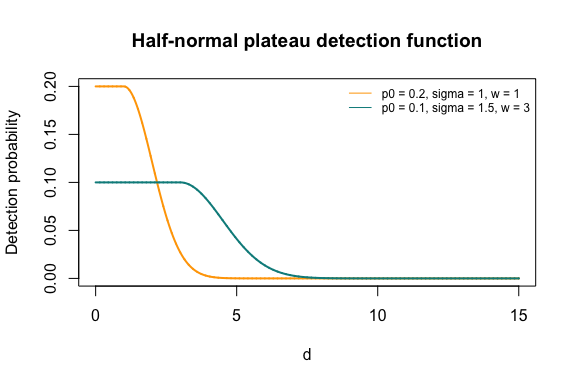The function ‘getHomeRangeArea()’ uses numerical approximation (to find the root) and estimate of $$95\%$$ home range radius and area for a set of circular detection functions available in nimbleSCR, viz., half-normal (detFun = 0), half-normal plateau (detFun = 1), exponential (detFun = 2), asymmetric logistic (detFun = 3), bimodal (detFun = 4), and donut (detFun = 5). The function ‘getHomeRangeArea()’ has two parts: a setup and and a run part. If we only want to calculate HR radius for a single set of parameter values, there is no need to compile. But for multiple sets of parameter values (e.g., an mcmc sample) one should compile the function before implementing the run part to reduce the computation time. ‘getHomeRangeArea()’ returns the estimates of home range radius and area for a given set of arguments with respect to a particular detection function using bisection algorithm: • Setup part • x: A vector or matrix (parameters in columns) of values for different parameters corresponding to the specified detection function. • xlim: A vector of length 2 giving the range along x-axis • ylim: A vector of length 2 giving the range along y-axis • nBreaks: A numeric variable denoting the number of breaks along an axis • tol: A numeric variable denoting the allowed tolerance in the radius estimate • nIter: A numeric variable giving the maximum number of iterations in bisection algorithm • detFun: A numeric variable denoting the type of detection function: 0 = Half-normal, 1 = Half-normal plateau, 2 = Exponential, 3 = Aysmmetric logistic, 4 = Bimodal, 5 = Donut. • prob: A numeric variable denoting the quantile probability to compute the radius • d: A numeric variable giving an initial value of the radius The run part of the function can be run without specifying any arguments. For more information on the detection functions, please refer to Dey et al. (2022). We provide some examples to compute home range radius and area in below. Next we obtain estimate $$95\%$$ home range radius and area for half-normal plateau detection function (detFun = 1). Note that, we only have to provide the parameter values for ‘sigma’ and ‘w’ (i.e., ‘p0’ is not needed) to compute home range radius and area. prob <- 0.95 paramnames.hr <- c("HRradius", "HRarea") params <- c(sigma, w) names(params) <- c("sigma", "w") HRAnim <- getHomeRangeArea( x = params, detFun = 1, prob = prob, d = 6, xlim = c(0, dim(habitatMask)), ylim = c(0, dim(habitatMask)), nBreaks = 800, tol = 1E-5, nIter = 2000) # Different values of argument "detFun" # 0 = Half-normal, 1 = Half-normal plateau, 2 = Exponential, # 3 = Aysmmetric logistic, 4 = Bimodal, 5 = Donut. HR.hnp <- c(HRAnimrun())
names(HR.hnp) <- paramnames.hr
print(HR.hnp)
##  HRradius    HRarea
##  3.546511 39.514144

Simulated values of $$95\%$$ home range radius and area are 3.55 du and 39.51 du$$^2$$, respectively.

We can also obtain estimates of home range size and its associated uncertainty from the MCMC chains of the parameters $$p_0, \sigma$$, and $$w$$.

myNimbleOutput <- as.mcmc.list(myNimbleOutput)
if(is.list(myNimbleOutput)){posteriorSamples <- do.call(rbind, myNimbleOutput)}
if(!is.list(myNimbleOutput)){posteriorSamples <- as.matrix(myNimbleOutput)}
theseIter <- round(seq(1, nrow(posteriorSamples), by = 10))
posteriorSamples <- posteriorSamples[theseIter,names(params)]
HRAnim.mat <- getHomeRangeArea(x = posteriorSamples, detFun = 1, prob = prob, d = 6,
nBreaks = 800, tol = 1E-5, nIter = 2000)

cHRAnim.arr <- compileNimble(HRAnim.mat, resetFunctions = TRUE)

HRA.Runtime <- system.time(
HR.chain <- cHRAnim.arrrun() ) print(HRA.Runtime) ## user system elapsed ## 49.364 0.096 49.603 dimnames(HR.chain)[] <- paramnames.hr From the obtained sample ‘HR.chain’ of $$95\%$$ home range radius and area, we can obtain summary statistics of these two metrics. HRest <- do.call(rbind, lapply(c(1:2), function(j){ c(mean(HR.chain[,j], na.rm = T), sd(HR.chain[,j], na.rm = T), quantile(HR.chain[,j], probs = c(0.025, 0.975), na.rm = T)) })) dimnames(HRest) <- list(paramnames.hr, c("MEAN", "SD", "2.5%", "97.5%")) cat("Numerical estimates using MCMC samples: \n", sep = "") ## Numerical estimates using MCMC samples: print(HRest) ## MEAN SD 2.5% 97.5% ## HRradius 3.558721 0.09277004 3.394315 3.756545 ## HRarea 39.813718 2.08249689 36.195473 44.332986 Based on the above analysis, the mean estimate of the $$95\%$$ home range radius is 3.56 du and the $$95\%$$ home range area is 39.81 du$$^2$$. For visual representation of the distributions of $$95\%$$ home range radius and area, we also get the traceplots and density of these metrics. HR.mcmc.sample <- as.mcmc.list(lapply(1:nchains, function(x) { niterPerChain <- nrow(HR.chain) / nchains theseRows <- ((x - 1) * niterPerChain + 1):(x * niterPerChain) this.MCMC.chain <- mcmc(HR.chain[theseRows, ]) return(this.MCMC.chain) })) names(HR.mcmc.sample) <- paste0("chain", 1:nchains) chainsPlot(HR.mcmc.sample, line = c(HR.hnp))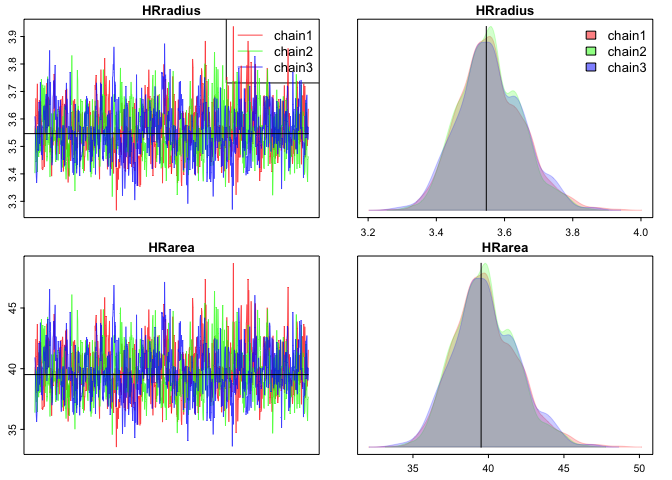The numerical estimate of home range radius and area can be improved further by tuning the function arguments: the number of bins (‘nBreaks’), the tolerance (‘tol’) and the uppler limit of the number of iterations for numerical approximation using bi-section algorithm (‘nIter’). However, tuning of these parameters will also affect the computation time of getHomeRangeArea(). ## 2. Calculating home range radius and area with other detection functions We can also fit SCR models with half-normal or exponential detection function using custom distributions such as ‘dbinomLocal_normal()’ or ‘dbinomLocal_exp()’, respectively. An example of SCR model fitting using half-normal detection and other efficient nimbleSCR features is given here. Fitting of SCR model using exponential detection function can be performed following similar steps (see ‘?dbinomLocal_exp’ for further details). Below, we provide examples to compute home range radius and area for the other detection functions available (Dey et al. 2022). ### Half-normal (detFun = 0) The half-normal detection function is written as: \begin{align} p_{\text{HN}}(d) = p_0 \, \exp\Big{(}-\frac{d^2}{2\sigma^2}\Big{)}, \end{align} where $$p_{0} \in (0,1)$$, $$\sigma > 0$$. Here $$p_0$$ denotes the baseline detection probability and $$\sigma$$ denotes the scale parameter. For a better understanding, we also plot the detection function against distance. ## HALF-NORMAL FUNCTION detfun.HN <- function(D, p0, sigma){ p0*exp(-D*D/(2*sigma*sigma))} par(mfrow=c(1,1)) x <- seq(0, 15, len=10001) plot(x, detfun.HN(D=x, p0=0.25, sigma = 2), type = "l", lwd=2, col = "orange", main = "Half-normal detection function", xlab = 'd', ylab = 'Detection probability') lines(x, detfun.HN(D=x, p0=0.15, sigma = 3), lwd=2, lty = "solid", col = "darkcyan") focal.vars <- c('p0', 'sigma') focal.values <- cbind(c(0.25, 0.15), # p0 c(2, 3)) # sigma legend.items <- apply(focal.values, 1, function(s){paste(paste(focal.vars,"=",s,sep=" "),collapse=", ")}) legend("topright", legend = legend.items, lty = c('solid', 'solid'), bg="transparent", col=c('orange', "darkcyan"), cex = 0.75, bty = 'n')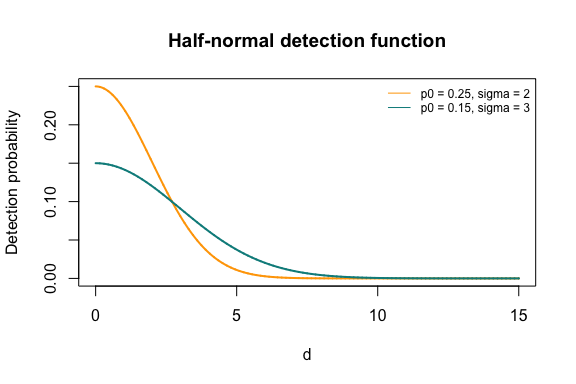Next we obtain the $$95\%$$ home range radius and area for half-normal detection function. Note that we only have to provide the parameter values for ‘sigma’ (i.e., ‘p0’ is not needed) to compute home range radius and area. # Half-normal sigma = 2 params <- c(sigma) names(params) <- c("sigma") HRAnim <- getHomeRangeArea(x = params, detFun = 0, prob = prob, d = 6, xlim = c(0, dim(habitatMask)), ylim = c(0, dim(habitatMask)), nBreaks = 800, tol = 1E-5, nIter = 2000) HR.hn <- c(HRAnimrun())
names(HR.hn) <- paramnames.hr
print(HR.hn)
##  HRradius    HRarea
##  4.897252 75.345054

### Exponential (detFun = 2)

The exponential detection function is written as: \begin{align} p_{\text{EXP}}(d) = p_0 \, \exp\Big{(}-\text{rate} \, * \, d\Big{)}, \end{align} where $$p_{0} \in (0,1)$$, $$\text{rate} > 0$$. Here $$p_0$$ denotes the baseline detection probability and $$\text{rate}$$ denotes the rate parameter. For a better understanding, we also plot the detection function against distance.

## EXPONENTIAL FUNCTION
detfun.EXP <- function(D, p0, rate){ p0*exp(-rate*D)}
par(mfrow=c(1,1))
x <- seq(0, 15, len=10001)
plot(x, detfun.EXP(D=x, p0=0.25, rate = 1/2), type = "l", lwd=2, col = "orange", main = "Exponential detection function", xlab = 'd', ylab = 'Detection probability')
lines(x, detfun.EXP(D=x, p0=0.15, rate = 1/3), lwd=2, lty = "solid", col = "darkcyan")
focal.vars <- c('p0', 'rate')
focal.values <- cbind(c(0.25, 0.15), # p0
c(1/2, round(1/3,2))) # rate

legend.items <- apply(focal.values, 1,
function(s){paste(paste(focal.vars,"=",s,sep=" "),collapse=", ")})
legend("topright",
legend = legend.items,
lty = c('solid', 'solid'),
bg="transparent",
col=c('orange', "darkcyan"),
cex = 0.75,
bty = 'n')